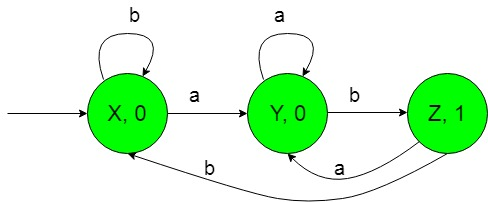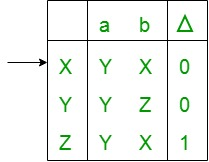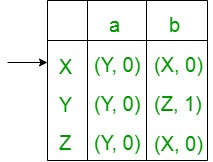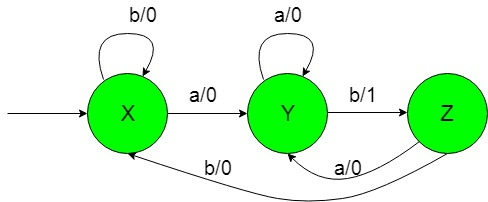# TOC | Moore and Mealy machines to count number of substring ‘ab’

Prerequisite: Mealy and Moore Machines, Difference between Mealy machine and Moore machine
Problem: Construction of the machines that take set of all string over {a, b} as input and count number of substring ‘ab’
Assume,

```Ε = {a, b} and
Δ = {0, 1} ```

where Ε and Δ are the input and output alphabet respectively.

Explanation:
The required Moore machine is constructed below.In the above diagram, the initial state ‘X’ on getting ‘b’ as the input it remains in the state of itself and print ‘0’ as the output and on getting ‘a’ as the input it transits to a state ‘Y’ and prints ‘0’ as the output.

The state ‘Y’ on getting ‘a’ as the input it remains in the state of itself and prints ‘0’ as the output and on getting ‘b’ as the input it transmits to the state ‘Z’ and prints ‘1’ as the output. The state ‘Z’ on getting ‘a’ as the input it transmits to the state ‘Y’ and prints ‘0’ as the output and on getting ‘b’ as the input it transmits to the state ‘X’ and prints ‘0’ as the output.

Thus finally above Moore machine can easily count the number of substring ‘ab’ i.e, on getting ‘ab’ as the input it gives ‘1’ as the output thus on counting the outputs ‘1’, we can easily count substring ‘ab’.

Conversion of Moore machine to Mealy machine:
Above Moore machine takes set of all string over {a, b} as input and prints ‘1’ as the output for every occurrence of ‘ab’ as substring. Now we need to transform the above transition diagram of Moore machine to equivalent Mealy machine transition diagram.

Steps for the required conversion are given below:

• Step-1: Formation of State Transition Table of the above Moore machine-In the above transition table, States ‘X’, ‘Y’ and ‘Z’ are kept in the first column which on getting ‘a’ as the input it transits to ‘Y’, ‘Y’ and ‘Y’ states respectively, kept in the second column and on getting ‘b’ as the input it transits to ‘X’, ‘Z’ and ‘X’ states respectively, kept in the third column. In the fourth column under Δ, there are corresponding outputs of the first column states. In the table, An arrow (→) indicates the initial state.

• Step-2: Formation of Transition Table for Mealy machine from above Transition Table of Moore machine-
Below transition table is going to be formed with the help of the above table and its entries just by using the corresponding output of the states of the first column and placing them in the second and third column accordingly.In the above table, the states in the first column like ‘X’ on getting ‘a’ as the input it goes to a state ‘Y’ and gives ‘0’ as the output and on getting ‘b’ as the input it goes to the state ‘X’ and gives ‘0’ as the output and so on for the remaining states in the first column. In the table, An arrow (→) indicates the initial state.

• Step-3: Then finally we can form the state transition diagram of Mealy machine with help of it’s above transition table.
The required diagram is shown below-Above Mealy machine takes set of all string over {a, b} as input and prints ‘1’ as the output for every occurrence of ‘ab’ as a substring.

Note: While converting from Moore to Mealy machine the number of states remains same for both Moore and Mealy machine but in case of Mealy to Moore conversion it do not give same number of states.My Personal Notes arrow_drop_upCheck out this Author's contributed articles.

If you like GeeksforGeeks and would like to contribute, you can also write an article using contribute.geeksforgeeks.org or mail your article to contribute@geeksforgeeks.org. See your article appearing on the GeeksforGeeks main page and help other Geeks.

Please Improve this article if you find anything incorrect by clicking on the "Improve Article" button below.

Article Tags :

Be the First to upvote.

Please write to us at contribute@geeksforgeeks.org to report any issue with the above content.